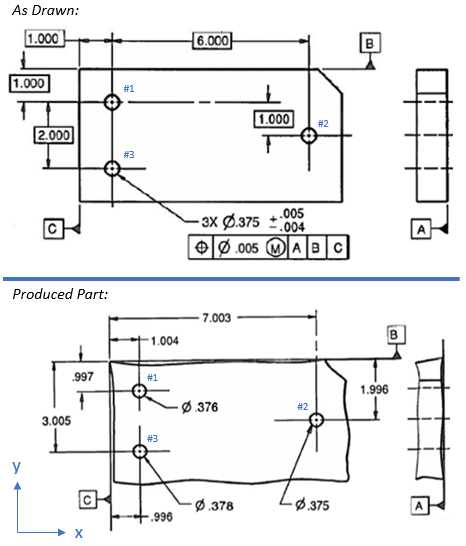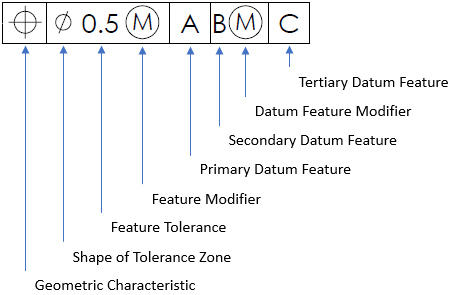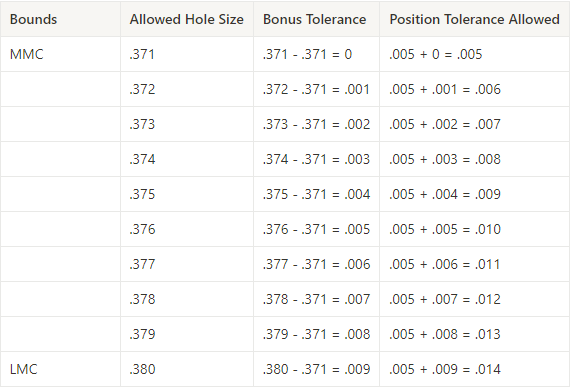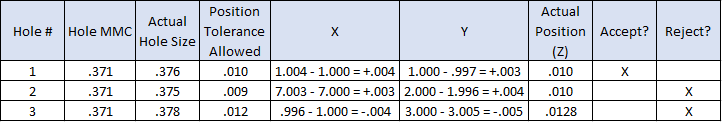## Accept or Reject?

Compare the produced part dimensions to the drawing requirements shown. Based on the positional tolerances, which hole number(s) would pass an inspection? Which hole number(s) would be rejected?Hint
Breaking down the general parts of a feature control frame:The drawing specifies a Max Material Condition (MMC) feature modifier for the .005 dia positional tolerance. A MMC modifier is the condition in which a feature of size contains the maximum amount of material within the stated limits of size. For a hole, MMC is the dimension that produces the smallest cutout. Thus,
$$MMC_{hole}=.375-.004=.371$$$Hint 2Breaking down the general parts of a feature control frame:The drawing specifies a Max Material Condition (MMC) feature modifier for the .005 dia positional tolerance. A MMC modifier is the condition in which a feature of size contains the maximum amount of material within the stated limits of size. For a hole, MMC is the dimension that produces the smallest cutout. Thus, $$MMC_{hole}=.375-.004=.371$$$
For a positional tolerance with a MMC feature modifier, there is a linearly increasing bonus tolerance as the actual hole size leans closer to LMC. LMC, or Least Material Condition, is the version of a feature that contains the least amount of material within the called out size limits. For a hole, LMC is the dimension that produces the largest cutout. Thus,
$$LMC_{hole}=.375+.005=.380$$$Now that the upper (LMC) and lower (MMC) bounds for hole size is determined, let’s calculate the bonus tolerances to find the total positional tolerance allowed for each hole size.To find the actual hole position on the produced part: $$Z=2\sqrt{X^2+Y^2}$$$
where $$X$$ is the difference between the x-coordinate hole position on the produced part and the drawing’s basic dimension associated with the hole’s x-direction, and $$Y$$ is the difference between the y-coordinate hole position on the produced part and the drawing’s basic dimension associated with the hole’s y-direction. Make sure to track +/- directions based on the established coordinate system.

A manufactured hole would be accepted/pass inspection if:
$$Z\leq Position\:Tolerance\:Allowed$$$A manufactured hole would be rejected/fail inspection if: $$Z> Position\:Tolerance\:Allowed$$$
Thus,#1: Accept
#2: Reject
#3: Reject